# Problems on VIDYA vs iVIDYA() Variable Index Dynamic Average65

Hello,

on my ExpertAdviros i call VIDYA indicator of metaquotes (in Indicator / examples folder) with iCustom () function

iVIDYA () which apparently uses another indicator code called "Variable Index Dynamic Average (in Indicator / Trend folder")

they are drawn on the chart with different values for some CMO-EMA parameters set... furthermore, by calling VIDYA () from my expert advisor, infinite values are returned on start (on firts bars) as if it were set to EMPTY_VALUE while it should be enhanced with drawable and calculable values

here an example

00:00:00 VIDY:179769313486231570814527423731704356798070567525844996598917476803157260780028538760589558632766878171540458953514382464234321326889464182768467546703537516986049910576551282076245490090389328944075868508455133942304583236903222948165808559332123348274797826204144723168738177180919299881250404026184124858368.000000 01:00:00 VIDYA:1.181070 IND2:0.000000 Balance: 100000.00 Equity: 100000.00

02:00:00 VIDYA:179493889086152583100140305077463104919191427039390112646610818293562895879462297752381587744491879511339330355864077654145734307655457917778866722107331011759117981721001653071841886350928074911377255641953719879726153780521235588603675532709066195164151667378333735157671790850689257124878413699429859065856.000000 03:00:00  VIDYA:179356396221604872993066084804886101655476746736257204232036761836559188643557217402664313383959791569093548862533162032968201882031340784950969949839700123150285568253885803779004965352693324322164984786606720417968752548566558794582481960146032022777996275518781016045071639942786438896661305769950269407232.000000

questions:

1) does anyone know why?

2) does anyone know where to find the indicator code which is in the default folder "Indicators / Trend" which in MT5 is called "Variable Index Dynamic Average"?

there should be no differences between the two indicators, they should coincide instead give different values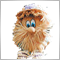308452

pablocode pablocode :65

here code on section

```OnInit()
.......
handle = iVIDyA(NULL,0,CmoPeriod,emaPeriod,0,price);   // use this for metaquotes "Variable Index Dynamic Average" on Indicator/Trend
handle = iCustom(NULL,0,"Examples\\VIDYAc",CmoPeriod,emaPeriod,0,price);   // use this for metaquotes VIDYA on Indicator/Examles
ArraySetAsSeries(Vidya1array,true);
.......```

and that on section

```OnTick()
.......
(CopyBuffer(handle,0,0,3,Vidya1array)>0) ..... take values of buffer
.......
```

it's simple like standard use, this soothfactor default

```//--- calculate smooth factor
ExtF=2.0/(1.0+InpPeriodEMA);```

but if i attach two indicators on chart only "Variable Index Dynamic Average" has infinite values before smooth instead VIDYA has empty_value

than smooth factor both has values but different for some CMO/EMA params set

it's strange not?!

1) have you an idea about?

2) second question,  while

VIDYA at  https://www.mql5.com/en/code/75

where can i find source code of  "Variable Index Dynamic Average" which is located Indicator/Trend (on MT5 indicators)?

thanks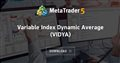Variable Index Dynamic Average (VIDYA)
• www.mql5.com
It is an original method of calculating the Exponential Moving Average (EMA) with the dynamically changing period of averaging. Period of averaging depends on the market volatility; as the measure of volatility Chande Momentum Oscillator (CMO) was chosen. This oscillator measures the ratio between the sum of positive increments and sum of...308452

pablocode :

here code on section

and that on section

it's simple like standard use, this soothfactor default

but if i attach two indicators on chart only "Variable Index Dynamic Average" has infinite values before smooth instead VIDYA has empty_value

than smooth factor both has values but different for some CMO/EMA params set

it's strange not?!

1) have you an idea about?

2) second question,  while

VIDYA at   https://www.mql5.com/en/code/75

where can i find source code of   "Variable Index Dynamic Average"  which is located Indicator/Trend (on MT5 indicators)?

thanks

Lots of mistakes.

1.'VIDYAc '- this indicator IS NOT SUPPLIED. Indicator 'VIDYA' supplied.

2. You have declared ONE variable 'handle' - but at the same time you want to store TWO HANDLES in this variable65

Lots of mistakes.

1.'VIDYAc '- this indicator IS NOT SUPPLIED. Indicator 'VIDYA' supplied.

2. You have declared ONE variable 'handle' - but at the same time you want to store TWO HANDLES in this variable

there is a little misunderstanding, no problem ..

handle was alternate (I reported both lines of code of the uses I made but only one line should be used depending on which flag to use)

i named VIDYAc it to make it clear that's called via iCustom but I am using VIDYA of the official metaquotes link  https://www.mql5.com/en/code/75

```OnInit ()
.......
handle = iVIDyA ( NULL , 0 , CmoPeriod, emaPeriod, 0 , prezzo);   // usalo per le metaquote "Media dinamica dell'indice variabile" su indicatore / indicatore di tendenza
handleCustom = iCustom ( NULL , 0 , "Esempi \\ VIDYA" , CmoPeriod, emaPeriod, 0 , prezzo);   // usa questo per metaquotes VIDYA su Indicator / Examles
ArraySetAsSeries (Vidya1array, true );
.......```

however, the problem also occurs on other few indicators called for example with iCustom or with the same native functions iXXX ..

at initial values the indicator has some correct values mixed with infinite values

please i'd like to repeat my two questions..

1) have you an idea about that?

2) second question,  where can i find source code of   "Variable Index Dynamic Average"  which is located Indicator/Trend (on MT5 indicators)?

thanksVariable Index Dynamic Average (VIDYA)
• www.mql5.com
It is an original method of calculating the Exponential Moving Average (EMA) with the dynamically changing period of averaging. Period of averaging depends on the market volatility; as the measure of volatility Chande Momentum Oscillator (CMO) was chosen. This oscillator measures the ratio between the sum of positive increments and sum of...308452

pablocode :

and indicator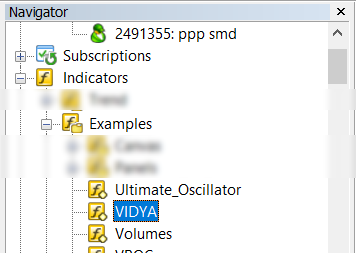are two identical indicators.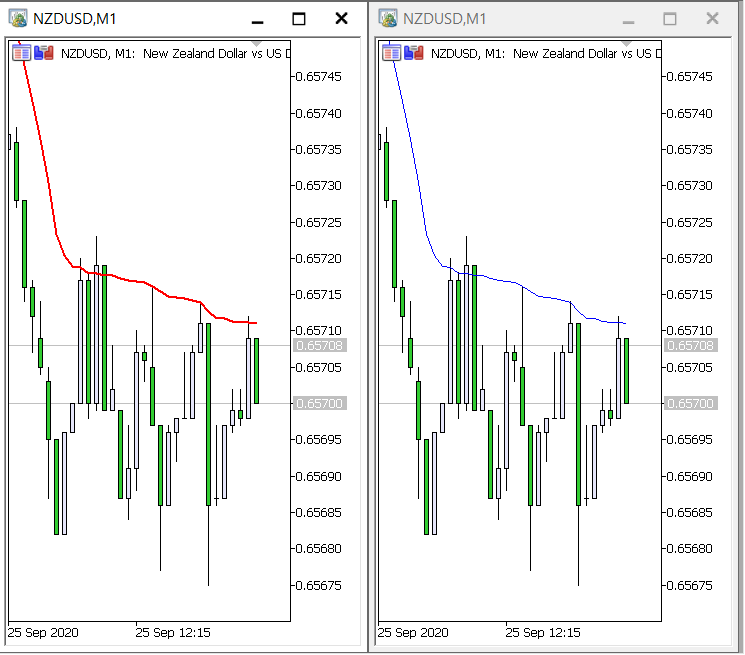308452

Code:

```//+------------------------------------------------------------------+
//|                                             iVIDyA Get Value.mq5 |
//+------------------------------------------------------------------+
#property version   "1.00"
//--- input parameters
input group                "VIDyA"
input int                 Inp_VIDyA_cmo_period     = 15;          // VIDyA: period for Chande Momentum
input int                 Inp_VIDyA_ema_period     = 12;          // VIDyA: EMA smoothing period
input int                 Inp_VIDyA_ma_shift       = 0;           // VIDyA: horizontal shift on the price chart
input ENUM_APPLIED_PRICE  Inp_VIDyA_applied_price  = PRICE_CLOSE; // VIDyA: type of price
//---
int      handle_iCustom;                       // variable for storing the handle of the iCustom indicator
int      handle_iVIDyA;                        // variable for storing the handle of the iVIDyA indicator
//+------------------------------------------------------------------+
//| Expert initialization function                                   |
//+------------------------------------------------------------------+
int OnInit()
{
//--- create handle of the indicator iCustom
handle_iCustom=iCustom(Symbol(),Period(),"Examples\\VIDYA",Inp_VIDyA_cmo_period,
Inp_VIDyA_ema_period,Inp_VIDyA_ma_shift,Inp_VIDyA_applied_price);
//--- if the handle is not created
if(handle_iCustom==INVALID_HANDLE)
{
//--- tell about the failure and output the error code
PrintFormat("Failed to create handle of the iCustom indicator for the symbol %s/%s, error code %d",
Symbol(),
EnumToString(Period()),
GetLastError());
//--- the indicator is stopped early
return(INIT_FAILED);
}
//--- create handle of the indicator
handle_iVIDyA=iVIDyA(Symbol(),Period(),Inp_VIDyA_cmo_period,
Inp_VIDyA_ema_period,Inp_VIDyA_ma_shift,Inp_VIDyA_applied_price);
//--- if the handle is not created
if(handle_iVIDyA==INVALID_HANDLE)
{
//--- tell about the failure and output the error code
PrintFormat("Failed to create handle of the iVIDyA indicator for the symbol %s/%s, error code %d",
Symbol(),
EnumToString(Period()),
GetLastError());
//--- the indicator is stopped early
return(INIT_FAILED);
}
//---
return(INIT_SUCCEEDED);
}
//+------------------------------------------------------------------+
//| Expert deinitialization function                                 |
//+------------------------------------------------------------------+
void OnDeinit(const int reason)
{
//---
if(handle_iCustom!=INVALID_HANDLE)
IndicatorRelease(handle_iCustom);
if(handle_iVIDyA!=INVALID_HANDLE)
IndicatorRelease(handle_iVIDyA);
}
//+------------------------------------------------------------------+
//| Expert tick function                                             |
//+------------------------------------------------------------------+
void OnTick()
{
//---
double custom[],vidya[];
ArraySetAsSeries(custom,true);
ArraySetAsSeries(vidya,true);
int start_pos=0,count=3;
if(!iGetArray(handle_iCustom,0,start_pos,count,custom))
return;
if(!iGetArray(handle_iVIDyA,0,start_pos,count,vidya))
return;
//---
string text="";
int limit=(count>3)?3:count;
for(int i=0; i<limit; i++)
{
text=text+
" bar #"+IntegerToString(i)+": "+
" iCustom "+DoubleToString(custom[i],Digits()+1)+
" iVIDyA "+DoubleToString(vidya[i],Digits()+1)+"\n";
}
Comment(text);
ChartRedraw(0);
}
//+------------------------------------------------------------------+
//| Get value of buffers                                             |
//+------------------------------------------------------------------+
bool iGetArray(const int handle,const int buffer,const int start_pos,
const int count,double &arr_buffer[])
{
bool result=true;
if(!ArrayIsDynamic(arr_buffer))
{
PrintFormat("ERROR! EA: %s, FUNCTION: %s, this a no dynamic array!",__FILE__,__FUNCTION__);
return(false);
}
ArrayFree(arr_buffer);
//--- reset error code
ResetLastError();
//--- fill a part of the iBands array with values from the indicator buffer
int copied=CopyBuffer(handle,buffer,start_pos,count,arr_buffer);
if(copied!=count)
{
//--- if the copying fails, tell the error code
PrintFormat("ERROR! EA: %s, FUNCTION: %s, amount to copy: %d, copied: %d, error code %d",
__FILE__,__FUNCTION__,count,copied,GetLastError());
//--- quit with zero result - it means that the indicator is considered as not calculated
return(false);
}
return(result);
}
//+------------------------------------------------------------------+
```

Result ( to play the gif animation, click on the picture )

Files:65

Code:

Result ( to play the gif animation, click on the picture )

# "MQL5 - HELP How to handle Custom indicator to different buffers of iEnvelopes (and others)?"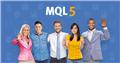MQL5 forum
• www.mql5.com
MQL5: Forum on automated trading systems and strategy testing65

Code:

Result ( to play the gif animation, click on the picture )

I find another behavior of the two indicators .. here is a video and a screenshot between "Variable Index Dynamic Average" and VIDYA,

taken from the points as indicated by you (which I already knew) infact I asked where I can find the mq5 source of "Variable Index Dynamic Media "not compiled ex5... because this issue it's very strange

however I attached them both indicators on the customized EURUSD chart from 2003 to 2019 (for optimizations)

but at the starts they give very different behaviors as I have already described (see also video)

so here the VIDYA used (instead i can't find source code for "Variable Index Dynamic Average" from Indicators/Trend but i think is the same)

```//+------------------------------------------------------------------+
//|                                                        VIDYA.mq5 |
//|                   Copyright 2009-2017, MetaQuotes Software Corp. |
//|                                              http://www.mql5.com |
//+------------------------------------------------------------------+
#property copyright   "2009-2017, MetaQuotes Software Corp."
#property description "Variable Index Dynamic Average"
//--- indicator settings
#property indicator_chart_window
#property indicator_buffers         1
#property indicator_plots           1
#property indicator_type1           DRAW_LINE
#property indicator_color1          Red
#property indicator_width1          1
#property indicator_label1          "VIDYA"
#property indicator_applied_price   PRICE_CLOSE
//--- input parameters
input int                InpPeriodCMO=9;              // Period CMO
input int                InpPeriodEMA=12;             // Period EMA
input int                InpShift=0;                  // Indicator's shift
//--- indicator buffers
double                  VIDYA_Buffer[];
//---
double                  ExtF;
//+------------------------------------------------------------------+
//| Custom indicator initialization function                         |
//+------------------------------------------------------------------+
void OnInit()
{
//--- indicator buffers mapping
SetIndexBuffer(0,VIDYA_Buffer,INDICATOR_DATA);
//--- sets first bar from what index will be drawn
PlotIndexSetInteger(0,PLOT_DRAW_BEGIN,InpPeriodEMA+InpPeriodCMO-1);
//--- sets indicator shift
PlotIndexSetInteger(0,PLOT_SHIFT,InpShift);
//--- name for indicator label
IndicatorSetString(INDICATOR_SHORTNAME,"VIDYA("+string(InpPeriodCMO)+","+string(InpPeriodEMA)+")");
//--- name for index label
PlotIndexSetString(0,PLOT_LABEL,"VIDYA("+string(InpPeriodCMO)+","+string(InpPeriodEMA)+")");
//--- calculate smooth factor
ExtF=2.0/(1.0+InpPeriodEMA);
//--- initialization done
}
//+------------------------------------------------------------------+
//| Variable Index Dynamic Average                                   |
//+------------------------------------------------------------------+
int OnCalculate(const int rates_total,
const int prev_calculated,
const int begin,
const double &price[])
{
//--- check for data
if(rates_total<InpPeriodEMA+InpPeriodCMO-1)
return(0);
//---
int limit;
if(prev_calculated<InpPeriodEMA+InpPeriodCMO-1)
{
limit=InpPeriodEMA+InpPeriodCMO-1;
for(int i=0;i<limit;i++)
VIDYA_Buffer[i]=price[i];
}
else limit=prev_calculated-1;
//--- main cycle
for(int i=limit;i<rates_total && !IsStopped();i++)
{
//--- calculate CMO and get absolute value
double mulCMO=fabs(CalculateCMO(i,InpPeriodCMO,price));
//--- calculate VIDYA
VIDYA_Buffer[i]=price[i]*ExtF*mulCMO+VIDYA_Buffer[i-1]*(1-ExtF*mulCMO);
}
//--- OnCalculate done. Return new prev_calculated.
return(rates_total);
}
//+------------------------------------------------------------------+
//| Chande Momentum Oscillator                                       |
//+------------------------------------------------------------------+
double CalculateCMO(int Position,const int PeriodCMO,const double &price[])
{
double resCMO=0.0;
double UpSum=0.0,DownSum=0.0;
if(Position>=PeriodCMO && ArrayRange(price,0)>Position)
{
for(int i=0;i<PeriodCMO;i++)
{
double diff=price[Position-i]-price[Position-i-1];
if(diff>0.0)
UpSum+=diff;
else
DownSum+=(-diff);
}
if(UpSum+DownSum!=0.0)
resCMO=(UpSum-DownSum)/(UpSum+DownSum);
}
return(resCMO);
}
//+------------------------------------------------------------------+
```

THIS ISSUE IS VERY STRANGE... HAVE YOU AN IDEA ABOUT?Variable Index Dynamic Average (VIDYA)
• www.mql5.com
It is an original method of calculating the Exponential Moving Average (EMA) with the dynamically changing period of averaging. Period of averaging depends on the market volatility; as the measure of volatility Chande Momentum Oscillator (CMO) was chosen. This oscillator measures the ratio between the sum of positive increments and sum of...
Files:308452

pablocode :

I don't understand your question. What do you dislike?65